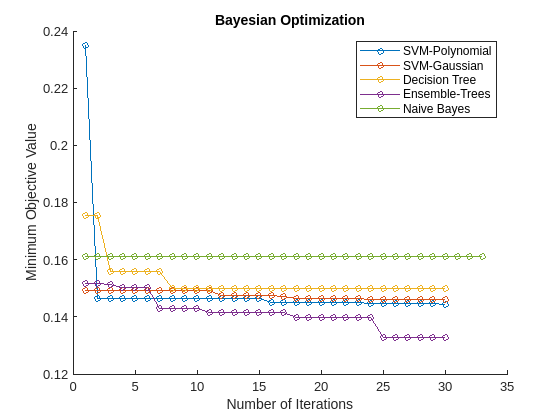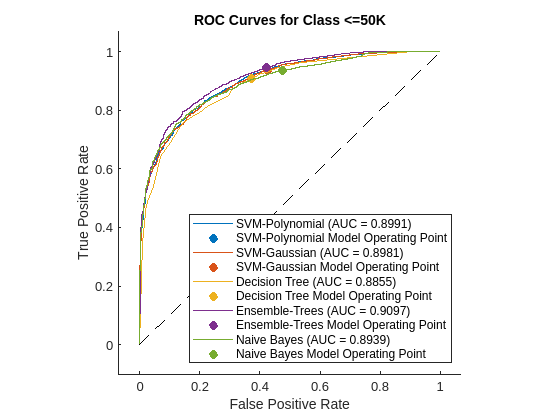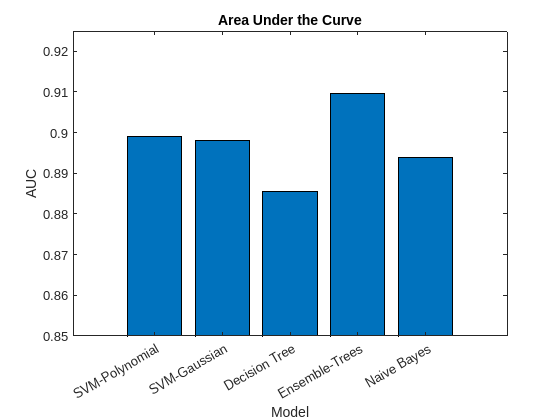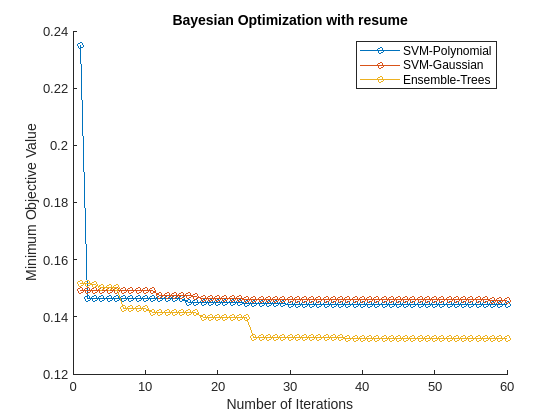# Moving Towards Automating Model Selection Using Bayesian Optimization

This example shows how to build multiple classification models for a given training data set, optimize their hyperparameters using Bayesian optimization, and select the model that performs the best on a test data set.

Training several models and tuning their hyperparameters can often take days or weeks. Creating a script to develop and compare multiple models automatically can be much faster. You can also use Bayesian optimization to speed up the process. Instead of training each model with different sets of hyperparameters, you select a few different models and tune their default hyperparameters using Bayesian optimization. Bayesian optimization finds an optimal set of hyperparameters for a given model by minimizing the objective function of the model. This optimization algorithm strategically selects new hyperparameters in each iteration and typically arrives at the optimal set of hyperparameters more quickly than a simple grid search. You can use the script in this example to train several classification models using Bayesian optimization for a given training data set and identify the model that performs best on a test data set.

Alternatively, to choose a classification model automatically across a selection of classifier types and hyperparameter values, use `fitcauto`. For an example, see Automated Classifier Selection with Bayesian and ASHA Optimization.

This example uses the 1994 census data stored in `census1994.mat`. The data set consists of demographic data from the US Census Bureau to predict whether an individual makes over \$50,000 per year. The classification task is to fit a model that predicts the salary category of people given their age, working class, education level, marital status, race, and so on.

Load the sample data `census1994` and display the variables in the data set.

```load census1994 whos```
``` Name Size Bytes Class Attributes Description 20x74 2960 char adultdata 32561x15 1872567 table adulttest 16281x15 944467 table ```

`census1994` contains the training data set `adultdata` and the test data set `adulttest`. For this example, to reduce the running time, subsample 5000 training and test observations each, from the original tables `adultdata` and `adulttest`, by using the `datasample` function. (You can skip this step if you want to use the complete data sets.)

```NumSamples = 5000; s = RandStream('mlfg6331_64'); % For reproducibility adultdata = datasample(s,adultdata,NumSamples,'Replace',false); adulttest = datasample(s,adulttest,NumSamples,'Replace',false);```

Preview the first few rows of the training data set.

`head(adultdata)`
```ans=8×15 table age workClass fnlwgt education education_num marital_status occupation relationship race sex capital_gain capital_loss hours_per_week native_country salary ___ ___________ __________ ____________ _____________ __________________ _________________ ______________ _____ ______ ____________ ____________ ______________ ______________ ______ 39 Private 4.91e+05 Bachelors 13 Never-married Exec-managerial Other-relative Black Male 0 0 45 United-States <=50K 25 Private 2.2022e+05 11th 7 Never-married Handlers-cleaners Own-child White Male 0 0 45 United-States <=50K 24 Private 2.2761e+05 10th 6 Divorced Handlers-cleaners Unmarried White Female 0 0 58 United-States <=50K 51 Private 1.7329e+05 HS-grad 9 Divorced Other-service Not-in-family White Female 0 0 40 United-States <=50K 54 Private 2.8029e+05 Some-college 10 Married-civ-spouse Sales Husband White Male 0 0 32 United-States <=50K 53 Federal-gov 39643 HS-grad 9 Widowed Exec-managerial Not-in-family White Female 0 0 58 United-States <=50K 52 Private 81859 HS-grad 9 Married-civ-spouse Machine-op-inspct Husband White Male 0 0 48 United-States >50K 37 Private 1.2429e+05 Some-college 10 Married-civ-spouse Adm-clerical Husband White Male 0 0 50 United-States <=50K ```

Each row represents the attributes of one adult, such as age, education, and occupation. The last column `salary` shows whether a person has a salary less than or equal to \$50,000 per year or greater than \$50,000 per year.

### Understand Data and Choose Classification Models

Statistics and Machine Learning Toolbox™ provides several options for classification, including classification trees, discriminant analysis, naive Bayes, nearest neighbors, support vector machines (SVMs), and classification ensembles. For the complete list of algorithms, see Classification.

Before choosing the algorithms to use for your problem, inspect your data set. The census data has several noteworthy characteristics:

• The data is tabular and contains both numeric and categorical variables.

• The data contains missing values.

• The response variable (`salary`) has two classes (binary classification).

Without making any assumptions or using prior knowledge of algorithms that you expect to work well on your data, you simply train all the algorithms that support tabular data and binary classification. Error-correcting output codes (ECOC) models are used for data with more than two classes. Discriminant analysis and nearest neighbor algorithms do not analyze data that contains both numeric and categorical variables. Therefore, the algorithms appropriate for this example are SVMs, a decision tree, an ensemble of decision trees, and a naive Bayes model.

### Build Models and Tune Hyperparameters

To speed up the process, customize the hyperparameter optimization options. Specify `'ShowPlots'` as `false` and `'Verbose'` as 0 to disable plot and message displays, respectively. Also, specify `'UseParallel'` as `true` to run Bayesian optimization in parallel, which requires Parallel Computing Toolbox™. Due to the nonreproducibility of parallel timing, parallel Bayesian optimization does not necessarily yield reproducible results.

`hypopts = struct('ShowPlots',false,'Verbose',0,'UseParallel',true);`

Start a parallel pool.

`poolobj = gcp;`

You can fit the training data set and tune parameters easily by calling each fitting function and setting its `'OptimizeHyperparameters'` name-value pair argument to `'auto'`. Create the classification models.

```% SVMs: SVM with polynomial kernel & SVM with Gaussian kernel mdls{1} = fitcsvm(adultdata,'salary','KernelFunction','polynomial','Standardize','on', ... 'OptimizeHyperparameters','auto','HyperparameterOptimizationOptions', hypopts); mdls{2} = fitcsvm(adultdata,'salary','KernelFunction','gaussian','Standardize','on', ... 'OptimizeHyperparameters','auto','HyperparameterOptimizationOptions', hypopts); % Decision tree mdls{3} = fitctree(adultdata,'salary', ... 'OptimizeHyperparameters','auto','HyperparameterOptimizationOptions', hypopts); % Ensemble of Decision trees mdls{4} = fitcensemble(adultdata,'salary','Learners','tree', ... 'OptimizeHyperparameters','auto','HyperparameterOptimizationOptions', hypopts); % Naive Bayes mdls{5} = fitcnb(adultdata,'salary', ... 'OptimizeHyperparameters','auto','HyperparameterOptimizationOptions', hypopts);```
```Warning: It is recommended that you first standardize all numeric predictors when optimizing the Naive Bayes 'Width' parameter. Ignore this warning if you have done that. ```

### Plot Minimum Objective Curves

Extract the Bayesian optimization results from each model and plot the minimum observed value of the objective function for each model over every iteration of the hyperparameter optimization. The objective function value corresponds to the misclassification rate measured by five-fold cross-validation using the training data set. The plot compares the performance of each model.

```figure hold on N = length(mdls); for i = 1:N mdl = mdls{i}; results = mdls{i}.HyperparameterOptimizationResults; plot(results.ObjectiveMinimumTrace,'Marker','o','MarkerSize',5); end names = {'SVM-Polynomial','SVM-Gaussian','Decision Tree','Ensemble-Trees','Naive Bayes'}; legend(names,'Location','northeast') title('Bayesian Optimization') xlabel('Number of Iterations') ylabel('Minimum Objective Value')```Using Bayesian optimization to find better hyperparameter sets improves the performance of models over several iterations. In this case, the plot indicates that the ensemble of decision trees has the best prediction accuracy for the data. This model performs well consistently over several iterations and different sets of Bayesian optimization hyperparameters.

### Check Performance with Test Set

Check the classifier performance with the test data set by using the confusion matrix and the receiver operating characteristic (ROC) curve.

Find the predicted labels and the score values of the test data set.

```label = cell(N,1); score = cell(N,1); for i = 1:N [label{i},score{i}] = predict(mdls{i},adulttest); end```

#### Confusion Matrix

Obtain the most likely class for each test observation by using the `predict` function of each model. Then compute the confusion matrix with the predicted classes and the known (true) classes of the test data set by using the `confusionchart` function.

```figure c = cell(N,1); for i = 1:N subplot(2,3,i) c{i} = confusionchart(adulttest.salary,label{i}); title(names{i}) end```The diagonal elements indicate the number of correctly classified instances of a given class. The off-diagonal elements are instances of misclassified observations.

#### ROC Curve

Inspect the classifier performance more closely by plotting an ROC curve for each classifier. Use the `perfcurve` function to obtain the `X` and `Y` coordinates of the ROC curve and the area under the curve (AUC) value for the computed `X` and `Y`.

To plot the ROC curves for the score values corresponding to the label `'<=50K'`, check the column order of the score values returned from the `predict` function. The column order is the same as the category order of the response variable in the training data set. Display the category order.

`c = categories(adultdata.salary)`
```c = 2×1 cell {'<=50K'} {'>50K' } ```

Plot the ROC curves.

```figure hold on AUC = zeros(1,N); for i = 1:N [X,Y,~,AUC(i)] = perfcurve(adulttest.salary,score{i}(:,1),'<=50K'); plot(X,Y) end title('ROC Curves') xlabel('False Positive Rate') ylabel('True Positive Rate') legend(names,'Location','southeast')```An ROC curve shows the true positive rate versus the false positive rate (or, sensitivity versus 1–specificity) for different thresholds of the classifier output.

Now plot the AUC values using a bar graph. For a perfect classifier, whose true positive rate is always 1 regardless of the thresholds, AUC = 1. For a classifier that randomly assigns observations to classes, AUC = 0.5. Larger AUC values indicate better classifier performance.

```figure bar(AUC) title('Area Under the Curve') xlabel('Model') ylabel('AUC') xticklabels(names) xtickangle(30) ylim([0.85,0.925])```Based on the confusion matrix and the AUC bar graph, the ensemble of decision trees and SVM models achieve better accuracy than the decision tree and naive Bayes models.

### Resume Optimization of Most Promising Models

Running Bayesian optimization on all models for further iterations can be computationally expensive. Instead, select a subset of models that have performed well so far and continue the optimization for 30 more iterations by using the `resume` function. Plot the minimum observed values of the objective function for each iteration of Bayesian optimization.

```figure hold on selectedMdls = mdls([1,2,4]); newresults = cell(1,length(selectedMdls)); for i = 1:length(selectedMdls) newresults{i} = resume(selectedMdls{i}.HyperparameterOptimizationResults,'MaxObjectiveEvaluations',30); plot(newresults{i}.ObjectiveMinimumTrace,'Marker','o','MarkerSize',5) end title('Bayesian Optimization with resume') xlabel('Number of Iterations') ylabel('Minimum Objective Value') legend({'SVM-Polynomial','SVM-Gaussian','Ensemble-Trees'},'Location','northeast')```The first 30 iterations correspond to the first round of Bayesian optimization. The next 30 iterations correspond to the results of the `resume` function. Resuming optimization is useful because the loss continues to reduce further after the first 30 iterations.